Guo, W., Hu, F., De, X., Hou, Z., Wang, Z., and Jiang, X. (2022). "Description of the instantaneous stress drop behavior of a cornstraw-potato residue mixture based on stress relaxation models," BioResources 17(3), 5255-5267.

#### Abstract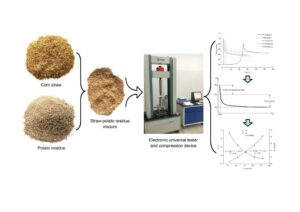Studying the stress relaxation characteristics can provide important process parameters for the granulation and briquetting of biomass materials. In this study, a generalized Maxwell model and fractional model were established to describe and analyze the stress instantaneous drop behavior of a cornstraw-potato residue mixture during the stress relaxation process. Stress relaxation characteristic parameters, e.g., the stress instantaneous drop time, were obtained. Using this information, the influence of the compression speed on the stress instantaneous drop time, stress relaxation time ratio, and other parameters were analyzed. In addition, the regression model of the compression frequency and compression speed was established, which provided a method for determining the appropriate compression frequency of the molding equipment according to the compression speed.

Description of the Instantaneous Stress Drop Behavior of a Cornstraw-Potato Residue Mixture Based on Stress Relaxation Models

Wenbin Guo, Fan Hu, Xuehong De,* Zhibo Hou, Zhipeng Wang, and Xinyang Jiang

Studying the stress relaxation characteristics can provide important process parameters for the granulation and briquetting of biomass materials. In this study, a generalized Maxwell model and fractional model were established to describe and analyze the stress instantaneous drop behavior of a cornstraw-potato residue mixture during the stress relaxation process. Stress relaxation characteristic parameters, e.g., the stress instantaneous drop time, were obtained. Using this information, the influence of the compression speed on the stress instantaneous drop time, stress relaxation time ratio, and other parameters were analyzed. In addition, the regression model of the compression frequency and compression speed was established, which provided a method for determining the appropriate compression frequency of the molding equipment according to the compression speed.

DOI: 10.15376/biores.17.3.5255-5267

Keywords: Cornstraw; Potato residue; Stress relaxation; Fractional model; Generalized Maxwell model

Contact information: Associate Professor; College of Mechanical and Electrical Engineering, Inner Mongolia Agricultural University, Hohhot 010018 China; *Corresponding author: dexuehong@126.com

GRAPHICAL ABSTRACTINTRODUCTION

As the residual waste from agricultural production, cornstraw and other viscoelastic materials are often crushed and compressed into feed pellets or fuel blocks for secondary use (Kaliyan and Morey 2009a; Kaliyan et al. 2009b; Rahaman and Salam 2017; Jewiarz et al. 2020). Stress relaxation is an important rheological characteristic for the compression process, which greatly affects the compression density, molding effect, and energy consumption of a material during granulation and briquetting. In order to analyze this impact, rheological models of viscoelastic materials have been established to describe their stress relaxation behavior, and the model parameters characterizing the stress relaxation behavior are obtained through nonlinear regression analysis (Peleg 1983; Fang et al. 2018; Joyner et al. 2021). Common stress relaxation models include the generalized Maxwell model (Mohsenin and Zaske 1976; Kelly 2011; Du et al. 2018), the Poynting Thomson Model (Yang 2010; Zhou et al. 2013), and the Zener Model (Chen et al. 2013; Yin et al. 2019). The model parameters generally include the stress relaxation time, modulus of elasticity, and viscosity coefficient. Among them, the stress relaxation time is often used to determine the compression frequency of the molding device, so as to avoid high stress areas and improve the performance of the molding equipment (Wang 2007).

As the compression rate and density of a material gradually increases during processing, a stress instantaneous drop stage appears during the stress relaxation process (Yang 2010). This stage does not last long, but the stress that occurs is large, which can easily cause large energy consumption and damage to the compression device. Therefore, it is necessary to find a suitable model to describe the stress drop process and obtain the stress dump time, so as to provide a basis for the reasonable selection of parameters , e.g., the compression frequency. Previous studies on the stress instantaneous drop during the stress relaxation are limited. For example, Wang (2007) and Yang (2010) studied the stress relaxation characteristics of forage grass and found that the generalized Maxwell model could not fit the curve at the stress drop phase in the case of few parameters. Thus, it is necessary to establish a more accurate model to describe this stress drop behavior. Since the speed of the stress relaxation is greatly affected by the compression speed, this study takes the stress relaxation characteristics of a cornstraw-potato residue mixture under different compression speeds as the research object (Ma 2015). By establishing a fractional model and a generalized Maxwell model, this study analyzes the stress instantaneous drop during the stress relaxation process and obtains the stress instantaneous drop time. Using this information, the relationship between the compression speed and the compression frequency is established.

EXPERIMENTAL

Materials

The corn stalks used in the test were selected from the whole plant that was naturally dried after harvesting in Hohhot, Inner Mongolia in autumn. The moisture content was (4% ± 0.1%) after being pulverized, and the scraps were sieved to a particle size of less than 3 mm using a punching sieve and then weighed. The potato waste residue was from a starch processing plant in Hohhot, Inner Mongolia, and its moisture content was (65% ± 1%) after pretreatment. In order to prevent material spoilage and reduce water loss, the potato waste residue was stored at a temperature of 2 to 4 °C. The relevant tests were completed within one week. The procedure was to mix cornstraw and potato residue in proportion, and then start the experiment. Referring to the research results of relevant mechanical tests (as found in Guo et al. (2022)), the cornstraw particles and potato residue were stirred and mixed at a mass ratio of 1 to 3. The sample of the cornstraw-potato residue mixture was obtained, as shown in Fig. 1.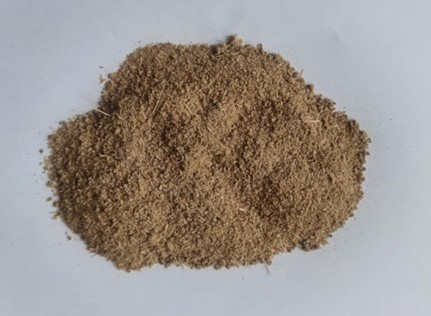Fig. 1. The mixture of cornstalk particle and potato residue

Experimental Method

The test equipment was a microcomputer-controlled electronic universal testing machine equipped with a self-made piston compression device (Fig. 2). The computer program controls the plunger for axial compression and pressure dwell and collects the pressure and displacement during the test. During the test, the cornstraw-potato residue mixture was compressed to a density of 900 kg/mm3 at different compression speeds (10, 60, 110, 160, and 210 mm/min). The dwell time of pressure was set to 900 s. After the test, the movable base plate was twitched to complete the extrusion of the mixed molding block. The test was repeated 3 times at the same compression speed at room temperature.

To analyze the relationship of the stress relaxation speed and the molding effect with the compression speed, the relaxation time ratio (Rt) and the relaxation ratio (Rr) were used as the test indicators in this study (Faborode and O’Callaghan 1986; O’Dogherty 1989; Ma et al. 2016). The Rt value is the ratio of the initial stress to the relaxation time for each stage of the stress relaxation process, which is calculated by Eq. 1,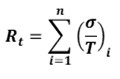(1)

where Rt is the stress relaxation time ratio (MPa/s), σ is the initial stress (MPa), T is the stress relaxation time (s), and is the stages of stress relaxation.

The Rr value is the ratio of the compression density (ρ) of the material in the stress relaxation test to the relaxed density (ρr) of the material block 24 h after demoulding. It can be calculated by Eq. 2,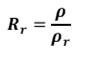(2)

where Rr is the relaxation ratio, ρ is the compression density (kg/m3), and ρr is the relaxation density (kg/m3). The smaller the relaxation ratio, the closer the ρ and ρr, and the better the molding effect of the material.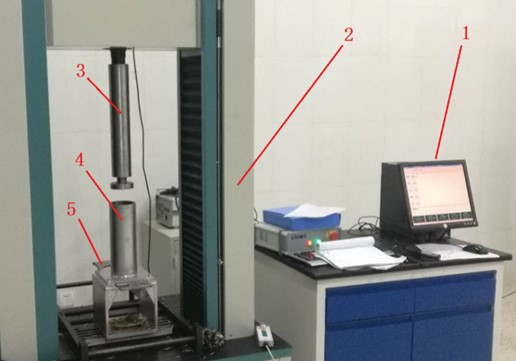Fig. 2. Electronic universal tester and compression device: 1. Computer; 2. Universal tester; 3. Plunger; 4. Compression device; and 5. Movable base plate

Constitutive Models

Generalized Maxwell model

Since the cornstraw-potato residue mixture is a viscoelastic material, the Three-element generalized Maxwell model was used to describe its stress relaxation behavior under different compression speeds and obtain parameters such as elastic modulus and stress relaxation time (Guo et al. 2022). This model is shown in Eq. 3,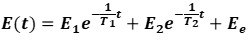(3)

where E1 and E2 are the decay moduli (MPa), Ee is the equilibrium modulus (MPa), and T1 and T2 are the decay time of stress. When t = 0, the initial elastic modulus of stress relaxation can be obtained, as shown in Eq. 4,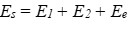(4)

The obtained results will be compared with the results of the fractional model to analyze the effect of the compression speeds on the stress relaxation characteristics of the cornstraw-potato residue mixture.

Fractional model

According to Hooke’s Law and Newton’s Law, the relationship between stress and strain during elastic and viscous deformation are shown as Eqs. 4 and 5, respectively,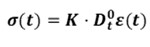(5)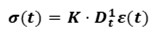(6)

where σ(t) in Eqs. 5 and 6 is the zero-order derivative () and the first-order derivative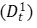of the strain ε(t) with respect to time (t), respectively, and K is the undetermined coefficient. The elastic deformation and viscous flow of the cornstraw-potato residue mixture appear simultaneously in the compression, and there is no obvious yield point, so the fractional order (β) is introduced to establish the fractional model of stress and strain, as shown in Eq. 7,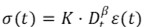(7)

where β is the fractional order whose value is between 0 and 1 (Stiassnie 1979; Nonnenmacher and Glockle 1991; Paola et al. 2011; Guo et al. 2017). According to the definition of a fractional order by the Riemann-Liouville derivative, Eq. 7 can be further rewritten as Eq. 8,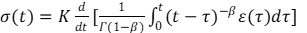(8)

where is the Gamma function, and is a dummy variable. Since the strain is constant during stress relaxation, the strain was replaced by a constant strain. Then, the variation of σ(t) over t is shown in Eq. 9,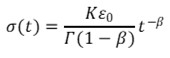(9)

After the left of Eq. 8 is transformed into the modulus E(t) at any time, the fractional model of stress relaxation obtained is shown in Eq. 10,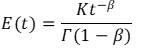(10)

According to rheological theory, stress relaxation is the phenomenon of stress attenuations that occurs when a material is instantly compressed to a constant density. However, due to the viscous resistance of the cornstraw-potato residue mixture, the stress generated by instantaneous compression tends to be infinite, which cannot be achieved in practice. Thus, the initial value of t in Eqs. 9 and 10 should be close but not equal to 0 (Koeller et al.1984). In order to accurately fit the stress relaxation curve of the cornstraw-potato residue mixture, the t in Eq. 10 is replaced by t + t0 to obtain the modified fractional model, as shown in Eq. 11,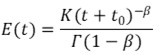(11)

where t0 is the actual start time of stress relaxation, and its magnitude is related to the compression speed during the test.

RESULTS AND DISCUSSION

Curve Analysis

Figure 3 shows the stress-time curves of the cornstraw-potato residue mixture at different compression speeds. It can be seen that before the cornstraw-potato residue mixture was compressed to a constant density of 900 kg/m3, the higher the compression speed, the faster the stress increased with time in the compression process, and the shorter the time and the larger the stress needed to reach a constant density. When the mixture was compressed to a constant density of 900 kg/m3 and the pressure started to dwell, the stress reached a peak (σ0) and began to decrease over time, showing obvious stress relaxation behavior. The decreasing stress trend at different compression speeds tended to be gentle as the time increased.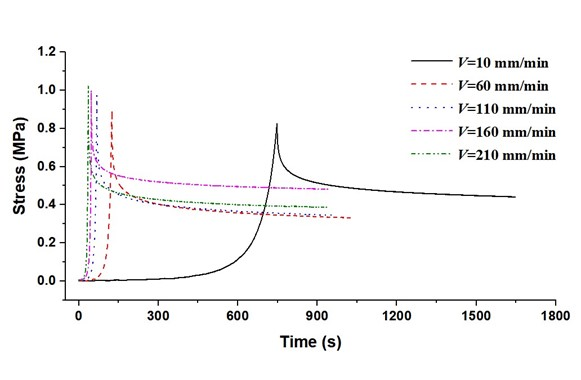Fig. 3. Stress-time curves of at different compression speeds

Model Evaluation and Analysis

In order to analyze the viscoelastic deformation and the stress dropping rate during the stress relaxation process of the cornstraw-potato residue mixture, the generalized Maxwell model was used for the fitting and regression analyses of the stress relaxation curves under different compression speeds. The results are shown in Table 1. The model fitting coefficient of determination (R2) was between 0.9832 to 0.9949. The larger the compression speed, the smaller the R2 and the worse the fitting effect. When the compression speed was 60, 110, 160, and 210 mm/min, R2 was below 0.99. The model curve did not fit the test curve well at the initial stage of stress decay. Consequently, the fitting effect was poor, and the fitting value could not reach the initial stress σ0, especially when the compression speed was high.

Table 1. Nonlinear Regression Analysis Results of Stress Relaxation Models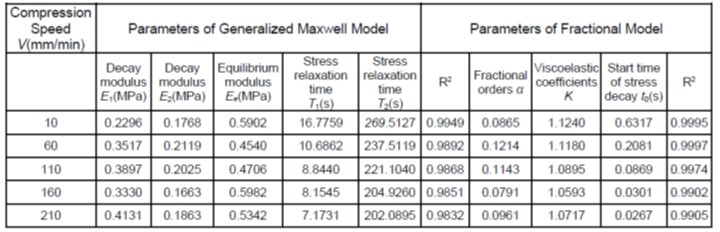It can be seen from the mechanical analogy of the generalized Maxwell model (Fig. 4a) that when the material was instantly compressed to a constant strain (constant density) in an ideal state, the resistance forces σ1, σ2, and σe were all from elastic deformation and could be obtained by the elastic modulus and constant strain. In practice, however, a viscoelastic material cannot be instantaneously compressed to a constant strain. For this reason, the instantaneous loading can only be replaced by loading at a certain speed, which results in the nonlinear viscoelastic deformation of the stress-time curve at the loading stage (Fig. 3). This means that when the viscoelastic material is compressed to a larger density at a certain speed, there will be some irrecoverable viscous deformation. According to Yang (2010), this viscous deformation behavior can be explained by adding a parallel damper element to the Zener Model, i.e., the Yang Model (as shown in Fig. 4b). The viscous deformation resistance (σ3) of the material during compression was partly from the damper element, and its magnitude was determined by the product of the viscous coefficient and the strain rate (Zhou 1994). Therefore, for materials with the same viscoelastic characteristics, the greater the compression speed, the greater the σ3, and the greater the initial stress (σ0) (σ0 = σ1 + σ2 + σ3) when the material reached constant density. Nevertheless, in the dwell stage, the plunger stopped moving, the viscous deformation resistance σ3 disappeared, and (σ0) instantaneously dropped (Yang et al. 2010). In this case, the larger the compression speed, the faster the stress σ0 declined at the beginning of the dwell stage (Fig. 3). Due to the abovementioned stress instantaneous drop stage in the stress relaxation process, the fitting accuracy of the generalized Maxwell model to the stress relaxation curve of the cornstraw-potato residue mixture was low. However, if the fitting accuracy was improved by increasing the number of elements in the generalized Maxwell model. This will not only complicate the model, but also it will increase the obtained parameters, making it difficult to analyze the stress relaxation characteristics of the cornstraw-potato residue mixture.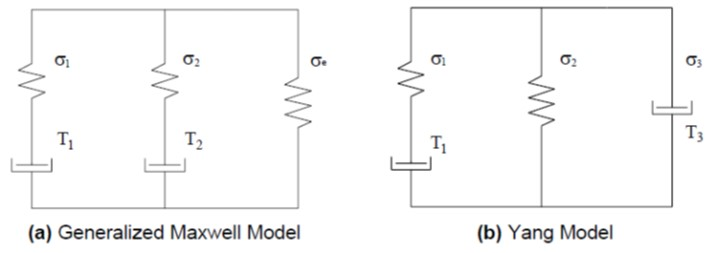Fig. 4. The mechanical analogy of the stress relaxation models

According to the stress relaxation characteristics of the cornstraw-potato residue mixture, the fractional model (as shown in Eq. 11) and the generalized Maxwell model were used to fit and analyze its stress relaxation curve (Fig. 5). Compared with the generalized Maxwell model, the fractional model can more accurately describe the entire stress relaxation process of the cornstraw-potato residue mixture, including the instantaneous drop stage of stress relaxation. In addition, as shown in Table 1, the fitting determination coefficient (R2) of the fractional model under different compression speeds was greater than 0.99, which was considerably better than the fitting results of the generalized Maxwell model. This was because the fractional model could accurately describe the viscoelastic deformation process during stress relaxation through the fractional order (Guo et al. 2017). In the obtained model parameters, t0 was the time when the stress began to instantaneously drop. The greater the compression speed (the closer it was to instantaneous compression), the smaller the t0 and the closer t0 was to 0, and the faster the stress relaxation response occurred after compression.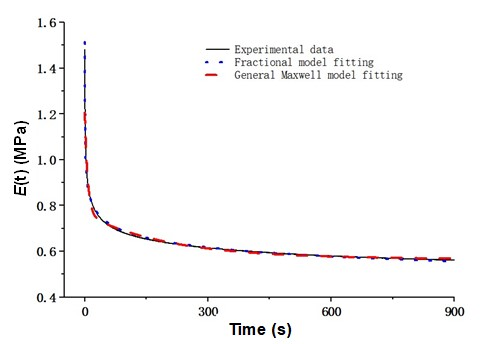Fig. 5. The stress relaxation curve fitted by different models

To compare the curve fitting results of the fractional model and the generalized Maxwell model, the stress relaxation process of the cornstraw-potato residue mixture was divided into the stress instantaneous drop stage and the stress decay stage, as shown in Fig. 6. The initial elastic modulus Es, as calculated by Eq. 12,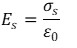(12)

where σs is the initial stress at the stress decay stage (MPa) and ε0 is the constant strain was obtained by fitting the stress relaxation curve with the generalized Maxwell model and was brought into Eq. 13, so the start time (ts) of the stress decay stage could be obtained. Based on ts, the time (Δt) for stress instantaneous drop can be obtained, as shown in Eq.14: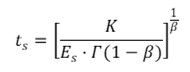(13)

With the obtained Δt, T1, and T2, the stress relaxation time ratio (Rt) can be calculated from Eq. 1,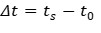(14)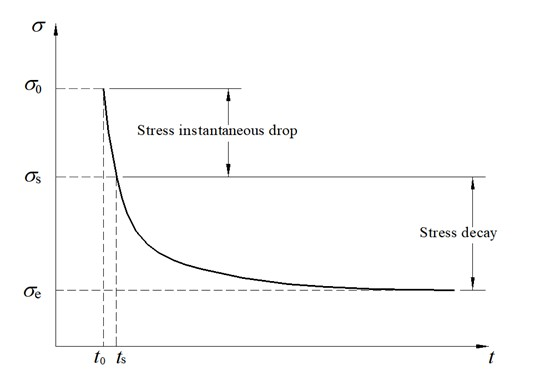Fig. 6. Stages of the stress relaxation

Effects of the Compression Speeds on the Stress Relaxation Parameters

To investigate the correlations of the parameters β, K, t0, and Δt (obtained at different compression speeds) with the stress relaxation speed and molding effect of the mixed material, correlation analysis was carried out on the following parameters: the relaxation time ratio (Rt), the relaxation ratio (Rr), and the generalized Maxwell model parameters (E1, E2, Ee, T1, and T2). The results are presented in Table 2. The compression speed V was significantly correlated with K, Δt, T1, T2, Rt, and Rr (significance level p-value less than or equal to 0.05). The compression speed was significantly negatively correlated with the Δt and T2, and significantly positively correlated with Rt (p-value less than or equal to 0.01). This indicated that the higher the compression speed, the faster the stress dropped during dwell, which in turn affected the relaxation ratio after dwell. Nonlinear regression analysis was performed on the Δt and Rt, which were significantly affected by the compression speed, and the determination coefficient (R2) was greater than 0.99 (Fig. 7). It can be seen that with the increase of V, the Δt and Rt decreased and increased exponentially, respectively, showing an obvious nonlinear variation law.

The Δt was significantly correlated with the t0, K, T1, T2, Rt, and Rr. Specifically, Δt had a significant positive correlation with t0, T1, and T2, which indicated that the earlier the stress started to plunge, the faster the stress plunged, and that the duration of the stress instantaneous drop directly impacted the stress decay time. In addition, the significant correlation between the Δt and the K, Rt, and Rr also demonstrated that in the case of a large compression rate, the stress instantaneous drop during stress relaxation was fast (Δt was small) and the relaxation time ratio was large. This indicated that the mixture particles were not well compacted, resulting in a large relaxation after demoulding and a poor molding effect. As for the fractional model parameters, K was significantly correlated with V, Δt, and T2, which partly reflected the impact of the compression speeds on the stress relaxation speed. Moreover, the change of the compression speed also impacted the degree of viscoelastic deformation of the mixture, and a smaller α indicated better elasticity of the material during the stress relaxation. Therefore, α was significantly negatively correlated with Ee. On the contrary, a larger α indicated better viscosity, thus α had a very significant positive correlation with E2.

Table 2. Correlation Analysis of Model Parameters and Compression Speed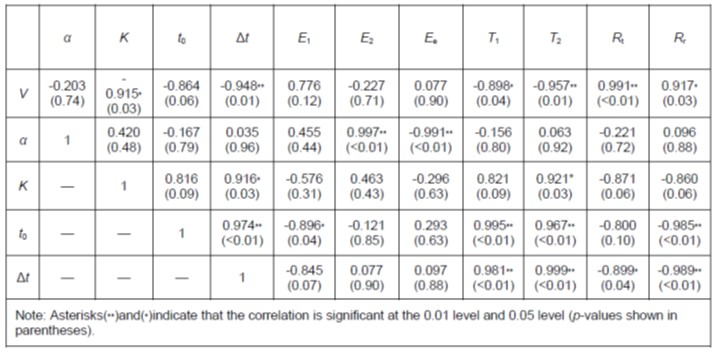To sum up, the compression speed (V) had an effect on the stress relaxation of the cornstraw-potato residue mixture. The larger the V, the shorter the stress instantaneous drop time and the stress decay time, and the faster the stress relaxation. Although the stress instantaneous drop time was short (Δt = 0.262 s to 1.472 s), the stress during which was the largest throughout the whole stress relaxation process, thereby exerting a negative impact on the molding energy consumption and the performance of the molding equipment during actual production. Therefore, when designing biomass molding equipment, e.g., granulators and briquetting machines, the compression time interval at different compression speeds can be determined by referring to the abovementioned calculation method of the stress instantaneous drop time (Δt), so as to avoid the highest stress ranges, reduce the energy consumption and equipment damage caused by large compressive stress, accurately control the compression frequency during the processing of biomass products, and improve the molding quality. Taking the cornstraw-potato residue mixture as an example, the relationship between the compression frequency (F) and the compression speed (V) was established by referring to the regression model, as shown in Fig. 7 and by Eq. 15,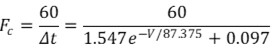(15)

where Fc is the maximum compression frequency (number of compressions/min), Δt is the compression time interval, i.e. the stress instantaneous drop time (s), and V is the compression speed (mm/min).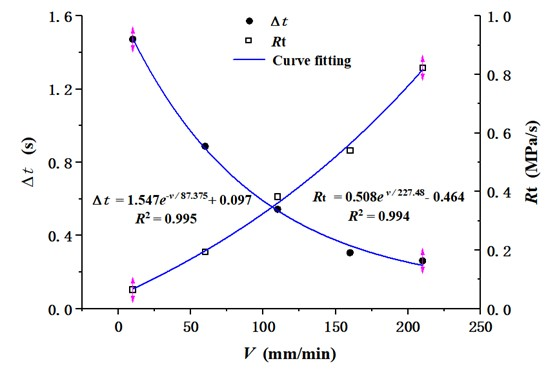Fig. 7. Nonlinear regression analysis

In actual production, the maximum compression frequency can be calculated according to the compression speed.

CONCLUSIONS

1. A fractional model was established to describe the stress instantaneous drop behavior during the stress relaxation process of a cornstraw-potato residue mixture and fit the stress relaxation curve. The determination coefficient (R2) was greater than 0.99. It was found that the stress relaxation process of the cornstraw-potato residue mixture could be divided into the stress instantaneous drop stage and the stress decay stage. The characteristic parameters affecting the stress relaxation speed, e.g., the stress instantaneous drop time (Δt) and the stress relaxation time ratio (Rt), were calculated. Furthermore, the reasons for the instantaneous stress drop during the cornstraw-potato residue mixture were analyzed.
2. The correlations between the compression speed (V) and the parameters of the stress relaxation model were obtained. A nonlinear regression analysis was carried out on the Δt and Rt, which were significantly correlated with the compression speed (V). Additionally, the change rule that Δt exponentially decreased with the increase of V was determined, and a regression model was established (R2 equals 0.995). On this basis, the relationship between the compression frequency (F) and the compression speed (V) was calculated. The result can provide a basis for determining the appropriate compression frequency when designing the molding equipment.

ACKNOWLEDGMENTS

The authors are grateful for the support from the National Natural Science Foundation of China (Grant No. 31960365 and Grant No. 51766016), the National key Research and Development Plan of China (Grant No. 2016YFD0701704), and the Research Program of Science and Technology at the Universities of Inner Mongolia Autonomous Region (Grant No. NJZY20045).

REFERENCES CITED

Chen, L., Liao, N., Xing, L., and Han, L. (2013). “Description of wheat straw relaxation behavior based on a fractional‐order constitutive model,” Agronomy Journal 105(1), 134-142. DOI: 10.2134/agronj2012.0190

Du, X., Wang, C., Guo, W., Wang, H., Jin, M., Liu, X., and Li, J. (2018). “Stress relaxation characteristics and influencing factors of sweet sorghum: Experimental study,” BioResources 13(4), 8761-8774. DOI: 10.15376/biores.13.4.8761-8774

Faborode, M. O., and O’Callaghan, J. R. (1986). “Theoretical analysis of the compression of fibrous agricultural materials,” Journal of Agricultural Engineering Research 35(3), 175-191. DOI: 10.1016/S0021-8634(86)80055-5

Fang, J., Zhang, Y., Yang, M., Wang, J., Liu, D., Gao, J., and Li, H. (2018). “Stress relaxation behavior and modeling of alfalfa during rotary compression,” Transactions of the Chinese Society of Agricultural Engineering 34(16), 50-56. DOI: 10.27229/d.cnki.gnmnu.2020.000066.

Guo, W., and Campanella, O. H. (2017). “A relaxation model based on the application of fractional calculus for describing the viscoelastic behavior of potato tubers,” Transactions of the ASAE American Society of Agricultural Engineers 60(1), 259-264. DOI: 10.13031/trans.11951.

Guo, W., Wang, Z., Hu, F., Hou, Z., and De, X. (2022). “Parameter optimization study based on co-briquetting tests of corn straw and potato residue,” BioResources 17(1), 1001-1014. DOI: 10.15376/biores.17.1.1001-1014.

Jewiarz, M., Mudryk. K., Wróbel, M., Fra˛czek, J., and Dziedzicet, K. (2020). “Parameters affecting RDF-based pellet quality,” Energies 13(4), 1-17. DOI: 10.3390/en13040910.0

Joyner, C. Z., Fernando, C., and Jaime, G. J. (2021). “Visco-elastic-plastic model to represent the compression behaviour of sugarcane agricultural residue,” Biosystems Engineering 212, 378-387. DOI: 10.1016/J.BIOSYSTEMSENG.2021.11.009

Kaliyan, N., and Morey, R. V. (2009a). “Constitutive model for densification of corn stover and switchgrass,” Biosystems Engineering 104(1), 47-63. DOI: org/10.1016/j.biosystemseng.2009.05.006.

Kaliyan, N., Morey, R. V., White, M. D., and Doering, A. (2009b). “Roll press briquetting and pelleting of corn stover and switchgrass,” Transactions of the ASABE 52(2), 543-555. DOI: 10.13031/2013.26812.

Kelly, P. A. (2011). “A viscoelastic model for the compaction of fibrous materials,” Journal of the Textile Institute 102(8), 689-699. DOI: 10.1080/00405000.2010.515103.

Koeller, R. C. (1984). “Applications of fractional calculus to the theory of viscoelasticity,” Journal of Applied Mechanics 51(2), 299-307. DOI: 10.1115/1.3167616

Ma, Y. (2015). “Study on rheological properties and product microstructure analysis in biomass densification under assistive vibration force field,” Inner Mongolia Agricultural University.

Ma, Y., Xuan, C., Wu, P., Yang, J., Su, H., and Zhang, Y. (2016). “Experiment on stress relaxation of corn stover during compression with assisted vibration,” Transactions of the Chinese Society of Agricultural Engineering 32(19), 88-94. DOI: 10.11975/j.issn.1002-6819.2016.19.012.

Mohsenin, N., and Zaske, J. (1976). “Stress relaxation and energy requirements in compaction of unconsolidated materials,” Journal of Agricultural Engineering Research 21(2), 193-205. DOI: 10.1016/0021-8634(76)90074-3.

Nonnenmacher, T. F., and Glockle, W. G. (1991). “A fractional model for mechanical stress relaxation,” Philosophical Magazine Letters 64(2), 89-93. DOI: 10.1080/09500839108214672.DOI:10.1016/S0307-904X(79)80063-3.

O’Dogherty, M. J. (1989). “A review of the mechanical behaviour of straw when compressed to high densities,” Journal of Agricultural Engineering Research 44(4), 241-265. DOI: 10.1016/S0021-8634(89)80086-1.

Paola, M. D., Pirrotta, A., and Valenza, A. (2011). “Visco-elastic behavior through fractional calculus: An easier method for best fitting experimental results,” Mechanics of Materials 43(12), 799-806. DOI: 10.1016/j.mechmat.2011.08.016.

Peleg, K. (1983). “A rheological model of nonlinear viscoplastic solids,” Journal of Rheology 27(5), 411-431. DOI: 10.1122/1.549714.

Rahaman, S. A., and Salam, P. A. (2017). “Characterization of cold densified rice straw briquettes and the potential use of sawdust as binder,” Fuel Processing Technology 158(Complete), 9-19. DOI: 10.1016/j.fuproc.2016.12.008.

Stiassnie, M. (1979). “On the application of fractional calculus for the formulation of viscoelastic models,” Applied Mathematical Modelling 3(4), 300-302. DOI: 10.1016/S0307-904X(79)80063-3

Wang, C. (2007). “Stress relaxation time of hay and its application,” Transactions of the Chinese Society for Agricultural Machinery 38(1), 65-67. DOI: 10.3969/j.issn.1000-1298.2007.01.016

Wang, H. (2007). “Study on the compressibility of Leymus chinensis and its stress relaxation characteristics analyzed by virtual prototyping technology,” Inner Mongolia Agricultural University. DOI: 10.7666/d.y1138182.

Yang, M., and Yang, H. F. (2010). “One special procedure in agricultural rheology and application of new relaxation model,” International Agriculture Engineering Conference 135-139. https://kns.cnki.net/kcms.

Yang, M. (2010). “Rheology of agricultural materials,” China Agricultural Press 20-36.

Yin, Y., Yang, Z., and Shi, M. (2019). “Complex plane analysis of fractional derivative model and its use for parameter determination of viscoelastic material,” Proceedings of 2019 2nd International Conference on Manufacturing Technology, Materials and Chemical Engineering 87-94. DOI: 10.1088/1757-899X/592/1/012009.

Zhou, Y., Chen, Y., and Chen, J. (2013). “Study of fractional Poynting-Thomson rheological model,” Journal of Southwest University of Science and Technology 28(1): 31-35. DOI: 10.3969/j.issn.1671-8755.2013.01.007.

Zhou, Z. (1994). “Agricultural materials science,” Beijing: China Agricultural Press.

Article submitted: June 11, 2022; Peer review completed: July 11, 2022; Revised version received and accepted: July 20, 2022; Published: July 26, 2022.

DOI: 10.15376/biores.17.3.5255-5267

ERRATUM: August 30, 2022: Figure 5 has been replaced with the correct version. The conclusions of this paper have not changed.